Abstract. John Sharp examines spirals and the Golden Section for the Nexus Network Journal, Winter 2002.

#Spirals and the Golden Section

John Sharp
20 The Glebe,Watford Herts
WD2 6LR, England

PART 1: TYPES OF SPIRALS

In order to understand different types of Golden Section spirals, it is necessary to be aware of the properties of different types of spirals. This section looks at spirals from that viewpoint.

CLARIFYING TYPES OF SPIRALS
A spiral is a plane curve that arises as a result of the movement of a point away from (or towards) a centre combined with a rotation about the centre. The centre is called the pole (Figure 1).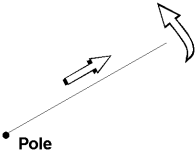Figure 1

The rules that relate the movement from the pole relative to the rotation affect the shape of the spiral. Many errors that arise in relation to descriptions of the spiral arise because of lack of appreciation of this fact.

Throughout this article r denotes the distance of a point on the spiral from the pole and q the angle of rotation of a line through the centre and that point. Different kinds of spirals are the result of the different ways that r can depend on q.

Archimedean spirals
Archimedean spirals are the simplest type of spiral. An everyday example is a coil of rope. The distinguishing feature of this type of spiral is that if you draw a line from the pole, the spiral cuts the line in equal divisions (Figure 2).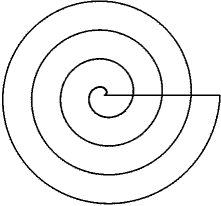Figure 2

It can be thought of as being based on addition or subtraction since the spiral is formed from the rule that for a given rotation angle (like one revolution) the distance from the pole has a fixed amount added.

The relationship between the movement and rotation is that the movement is directly proportional to the angle of rotation. Thus the dependence of r on q is given by

r = aq,

where a is the constant of proportionality. The multiplication of q by a is due to repeatedly adding the aforementioned fixed amount. For example, if q is measured simply in revolutions, then the fixed amount is simply a: after one revolution the distance from the pole, r, will be a; after two revolutions, r will be 2a; etc. It may be more convenient to measure in degrees (where one revolution corresponds to 360°) or radians (where one revolution corresponds to 2p radians), but the same reasoning still applies: multiplication is the result of repeated addition.

Different values of the constant a give Archimedean spirals that are tightly wound (small a) or loosely wound (large a) (Figure 3).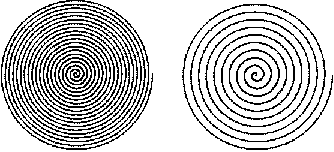Figure 3

If you magnify one of these spirals the correct amount, it will fit directly on the other one without any other movement like rotation. This is an important property that needs to be remembered as a contrast to the logarithmic (equiangular) spiral described below. Another important property is that the pole is a point on the spiral and it is easy to draw that point.

This spiral has nothing whatsoever to do with the Golden Section. The constant of proportionality a does not confer any special properties on the spiral. There is only one Archimedean spiral.

The logarithmic or equiangular spiral
This spiral can be thought of as being based on multiplication or division since the spiral is formed from the rule that for a given rotation angle (like one revolution) the distance from the pole is multiplied by a fixed amount. A special case of this multiplication factor is when it is the Golden Section f (or powers thereof) (Figure 4).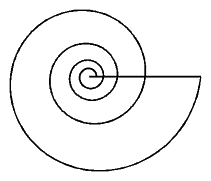Figure 4

The relationship between the movement and rotation is more complicated than the Archimedean spiral. As the line rotates so the distance of the point increases exponentially (due to repeated multiplication instead of repeated addition). In this case, the dependence of r on q is given by

r = abq,

with q measured in revolutions, or alternatively

r = aeq cot a,

with q measured in radians, where the constant a is a simple magnification factor, the number e is a universal constant (like p is), specifically the base of the natural logarithm (e » 2.718), and a is a constant angle. This could be written more simply as r = aecq by setting c = cot a, but that would not explicitly show a.

The reason to make a explicit is the following important property of the logarithmic spiral: a line from the pole of a logarithmic spiral makes a constant angle with the tangent to the spiral, namely a. Hence it is often called the equiangular spiral (Figure 5).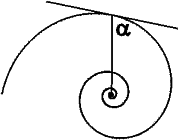Figure 5

The mathematical relationships between a and the aforementioned multiplication factor will be further examined and exploited in Part 3 and Part 4. For now, suffice it to note that different values of the constant a simply magnify the spiral, but different values of a determine the shape of the spiral. That is, there are an infinite number of logarithmic spirals, one for each choice of a. Conversely, given a member of the 'family' of logarithmic spirals, sufficient information about the particular spiral will allow you to determine the corresponding a. This will be done with a ubiquitous spiral based on the Golden Section, in Part 3.

By considering the spiral in terms of the tangent angle a, two curves can be seen as part of the family of logarithmic spirals. When a is 0° or 180° the spiral becomes a straight line, and when it is 90° it becomes a circle. This latter result is important when considering the Golden Section spiral produced approximately with arcs of circles. In characterising various Golden Section spirals, the tangent angle will be used to compare spirals produced in different ways.

If you simply magnify a logarithmic spiral, it will not fit on top of itself, but if you then rotate it about the pole an appropriate amount, it will. It is this property that is important for any logarithmic spiral, but it is especially important for the Golden Section logarithmic spiral. This is one point of contrast with the Archimedean spiral, the other being that it is not possible to draw the curve all the way to the pole since you need infinite division to reach it.

APPROXIMATIONS TO SPIRALS
Because of the way Golden Section spirals are often drawn, it is important to be aware that some logarithmic spirals can be drawn approximately. This usually means drawing them using arcs of circles.

For curves made up of portions of other curves to 'flow' without kinks or cusps, the tangents of two curves at the intersection point must be the same line. For two circles to join as if they are one curve, the intersection point must be on a line through their centres, so two arcs can be made to appear as if they are one continuous line as follows (Figure 6).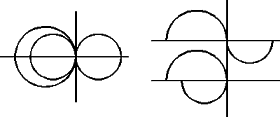Figure 6

Such a 'piecewise' curve cannot be described by a single mathematical equation, and, as we shall see when we draw a Golden Section logarithmic spiral using this technique, it can lead to properties which are different from the those of single-equation curves. Note that the circles do not have to be the same size. As long as the line joining the centres goes though the intersection point, the curve will appear smooth.

SPIRAL SIMILARITY
There is another aspect of spirals that is important, particularly because of the property of logarithmic spirals in which a combination of rotation and magnification allows the curve to be superimposed on itself. If this transformation is carried out on a motif, with a suitably matched rotation and magnification, a pattern can be produced which is comparable to frieze patterns or wallpaper patterns. The following figure gives an example (Figure 7).Figure 7

Note that although this uses rectangles, it has nothing to do with the Golden Section, although the techniques described in Parts 2 and 3 for producing a Golden Section spiral use a similar drawing. There are two types of rectangles in Figure 7, with sides in the ratio 4:3 and 12:7 (the latter being the ones containing the flies).

| Introduction | Part 1 | Part 2 | Part 3 | Part 4 |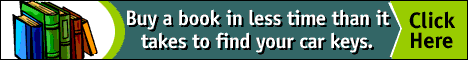top of page

NNJ Homepage

NNJ Winter 2002 Index

Order books!

Research Articles

The Geometer's Angle

Didactics

Book Reviews

Conference and Exhibit Reports

The Virtual Library

Submission Guidelines

Top of Page